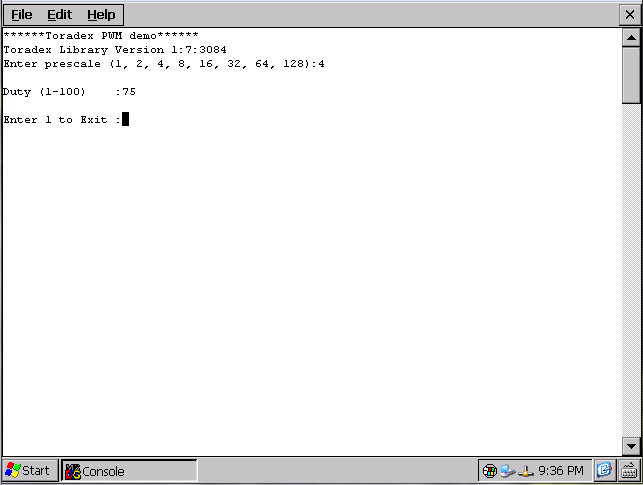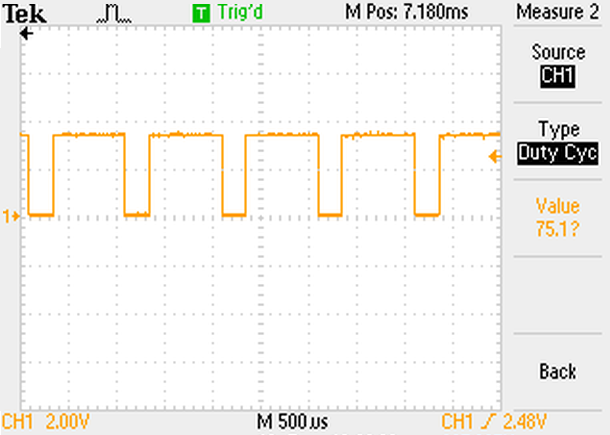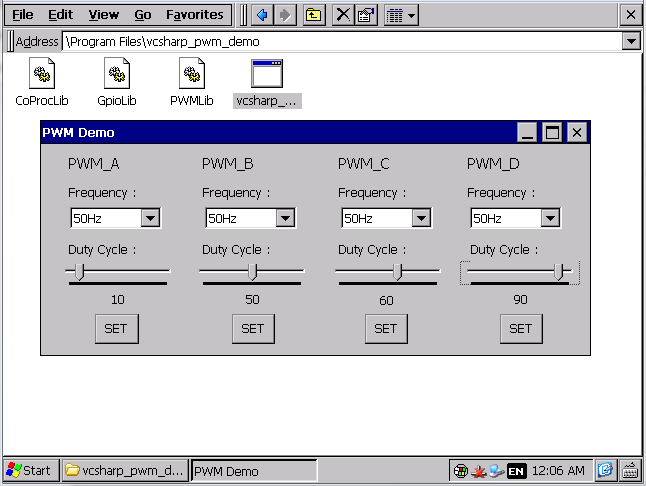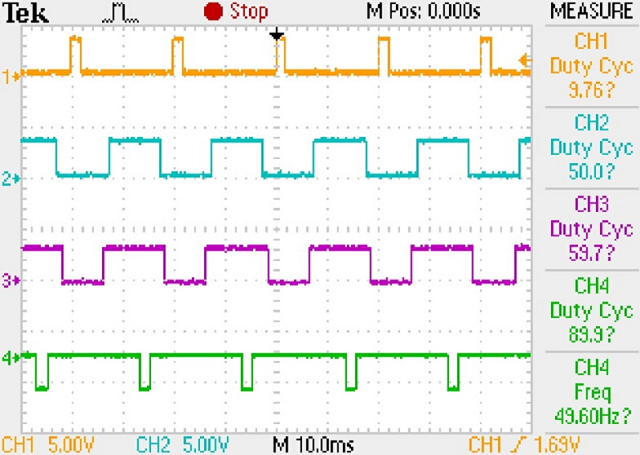# How to use PWM Library

danger

this is a legacy library and thus not supported by Toradex anymore. We recommend that you use the new libraries for all Toradex modules. Please see the Toradex CE Libraries and Code Samples for up-to-date information.

PWM library is used to generate PWM output on Toradex module.

Use cases:

• LCD backlight control
• Servo motor control
• and many more..

#### PWM Library​

We provide free Windows CE library for PWM including demo source code here.

VS2008 ProjectVS2013 ProjectVS2015 ProjectModule compatibilityLanguage
PWM--PXA / TegraC
PWMPWMPWMTegra / VFxx / iMX6C
PWM--PXA / TegraC#
PWM--VFxx / iMX6C#
info

PWM Channel 1 for PXA3xx (PXA320, PXA310, PXA300) is available on multiplexed GPIO pin of SODIMM Pin 152.
On Colibri Evaluation Board V3.1A, SODIMM Pin 152 is available at B7 of X3 (User extension).
On Iris Carrier Board V1.1, SODIMM Pin 152 is connected to PWM_D line through a not DNP 0E resistor (R110). Please refer Iris schematic.
To access the PWM channel 1 available on the multiplexed GPIO pin, set the alternate function of **other** GPIO to 0x07 (tristate).
On VFxx PWM1/PWM_A and PWM3/PWM_C is using same PWM controller i.e FTM controller 0 and PWM2/PWM_B and PWM4/PWM_D using FTM controller 1 due to this configuring PWM 1 result same configuration for PWM3 similarly for PWM 2 and PWM 4.
info

It is always recommended to update the libraries first before compiling the demo project. Click on this link to get latest libraries.

#### Demo application in C​

This application demonstrates the use of PWM library to generate PWM signal of user-defined frequency and duty cycle.Output wave 1000 Hz at 75% duty cycle#### Demo application in C#​Output waveform#### Calculations​

Frequency calculation for Colibri T30:

``Frequency (Hz) = 352100/Prescale Prescale range: 1 - 8192, Period = 256 (Fixed) Minimum frequency = 42.98 Hz (prescale = 8192) Maximum frequency = 352.10 kHz (prescale = 1)``

Frequency calculation for Colibri T20:

``Frequency (Hz) = 188700/Prescale Prescale range: 1 - 8192, Period = 256 (Fixed) Minimum frequency = 23.03 Hz (prescale = 8192) Maximum frequency = 188.70 kHz (prescale = 1)``

Frequency calculation for Colibri iMX6x:

``Frequency (Hz) = 66000000/(prescale x period) Prescale range: 1-4096, Period = 1 - 65536 Minimum frequency = 0.245 Hz (prescale = 128, period = 65536) Maximum frequency = 66 MHz (prescale = 1, period = 1)``

Frequency calculation for Colibri VF61:

``Frequency (Hz) = 83300000/(prescale x period) Prescale range: 1, 2, 4, 8, 16, 32, 64, 128, Period = 1 - 65536 Minimum frequency = 9.930 Hz (prescale = 128, period = 65536) Maximum frequency = 83.3 MHz (prescale = 1, period = 1)``

Frequency calculation for Colibri VF50:

``Frequency (Hz) = 66000000/(prescale x period) Prescale range: 1, 2, 4, 8, 16, 32, 64, 128, Period = 1 - 65536 Minimum frequency = 7.86 Hz (prescale = 128, period = 65536) Maximum frequency = 66 MHz (prescale = 1, period = 1)``

Frequency calculation for PXAxxx:

``Frequency (Hz) = 10^9/(76.9 x prescale x period) Prescale range: 1 - 64, Period = 1 - 1024 Minimum frequency = 198.42 Hz (prescale = 64, period = 1024) Maximum frequency = 3.25 MHz (prescale = 1, period = 4)``

Send Feedback!